How to Calculate and Solve for the Radius of Investigation in Well Testing | The Calculator Encyclopedia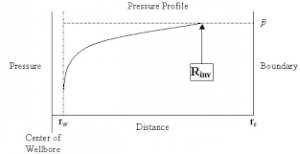The image above represents radius of investigation.

To compute for the radius of investigation, five essential parameters are needed and these parameters are permeability (k), porosity (φ), viscosity (μ), time (t) and total compressibility (CT).

The formula for calculating the radius of investigation:

rinv = 0.0325 √[Kt / φμCT]

Where:

rinv = Radius of Investigation
K = Permeability
φ = Porosity
μ = Viscosity
t = Time
CT = Total Compressibility

Let’s solve an example;
Find the radius of investigation when the permeability is 25, porosity is 18, viscosity is 12, time is 22 and total compressibility is 37.

This implies that;

K = Permeability = 25
φ = Porosity =18
μ = Viscosity = 12
t = Time = 22
CT = Total Compressibility = 37

rinv = 0.0325 √[Kt / φμCT]
rinv = 0.0325 √[25 x 22 / 18 x 12 x 37]
rinv = 0.0325 √[25 x 22 / 7992]
rinv = 0.0325 √[550 / 7992]
rinv = 0.0325 √[0.068]
rinv = 0.0325 [0.26]
rinv = 0.0085

Therefore, the radius of investigation is 0.0085 ft.

How to Calculate and Solve for Infinite Acting Period | The Calculator Encyclopedia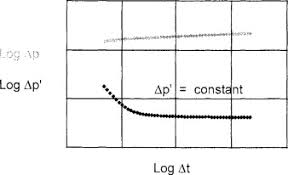The image represents the infinite acting period.

To compute the infinite acting period, six essential parameters are needed and these parameters are permeability (k), porosity (φ), well drainage area (A), viscosity (μ), Dimensionless Time to End of Infinite Acting Period ((tDA)eia) and total compressibility (CT).

The formula for calculating the infinite acting period:

teia = [φ μ CT A / 0.000263K] (tDA)eia

Where;

teia = Infinite Acting Period
K = Permeability
φ = Porosity
A = Well Drainage Area
μ = Viscosity
(tDA)eia = Dimensionless Time to End of Infinite Acting Period
CT = Total Compressibility

Let’s solve an example;
Given that the permeability is 21, porosity is 15, well drainage area is 32, viscosity is 26, dimensionless time to end of infinite acting period is 44 and total compressibility is 34.
Find the infinite acting period?

This implies that;

K = Permeability = 21
φ = Porosity = 15
A = Well Drainage Area = 32
μ = Viscosity = 26
(tDA)eia = Dimensionless Time to End of Infinite Acting Period = 44
CT = Total Compressibility = 34

teia = [φ μ CT A / 0.000263K] (tDA)eia
teia = [15 x 26 x 34 x 32 / 0.000263 x 21] 44
teia = [424320 / 0.005523] 44
teia = [76827810.972] 44
teia = 3380423682.78

Therefore, the infinite acting period is 3380423682.78 s.

How to Calculate and Solve for Dimensionless Time in Well Testing | The Calculator Encyclopedia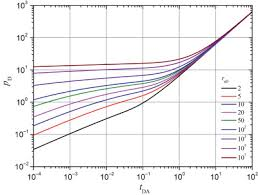The image above represents dimensionless time.

To compute for the dimensionless time, six essential parameters are needed and these parameters are permeability (k), porosity (φ), viscosity (μ), well radius (rw), minimum transient time (t) and total compressibility (CT).

The formula for calculating dimensionless time:

tD = 0.000263kt / φμCTr

Where;

tD = Dimensionless Time
k = Permeability
φ = Porosity
μ = Viscosity
rw = Well Radius
t = Minimum Transient Time
CT = Total Compressibility

Let’s solve an example
Find the dimensionless time when the permeability is 13, porosity is 11.5, viscosity is 10.5, well radius is 24, minimum transient time is 17 with a total compressibility of 28.

This implies that;

k = Permeability = 13
φ = Porosity = 11.5
μ = Viscosity = 10.5
rw = Well Radius = 24
t = Minimum Transient Time = 17
CT = Total Compressibility = 28

tD = 0.000263kt / φμCTr
tD = 0.000263 x 13 x 1711.5 x 10.5 x 28 x 24²
tD = 0.000263 x 13 x 1711.5 x 10.5 x 28 x 576
tD = 0.000263 x 13 x 171947456
tD = 0.0581231947456
tD = 2.9845e-8

Therefore, the dimensionless time is 2.98

Nickzom Calculator – The Calculator Encyclopedia is capable of calculating the dimensionless time.

To get the answer and workings of the dimensionless time using the Nickzom Calculator – The Calculator Encyclopedia. First, you need to obtain the app.

You can get this app via any of these means:

To get access to the professional version via web, you need to register and subscribe for NGN 1,500 per annum to have utter access to all functionalities.
You can also try the demo version via https://www.nickzom.org/calculator

Android (Paid) – https://play.google.com/store/apps/details?id=org.nickzom.nickzomcalculator
Android (Free) – https://play.google.com/store/apps/details?id=com.nickzom.nickzomcalculator
Apple (Paid) – https://itunes.apple.com/us/app/nickzom-calculator/id1331162702?mt=8
Once, you have obtained the calculator encyclopedia app, proceed to the Calculator Map, then click on Petroleum under EngineeringNow, Click on Well Testing under Petroleum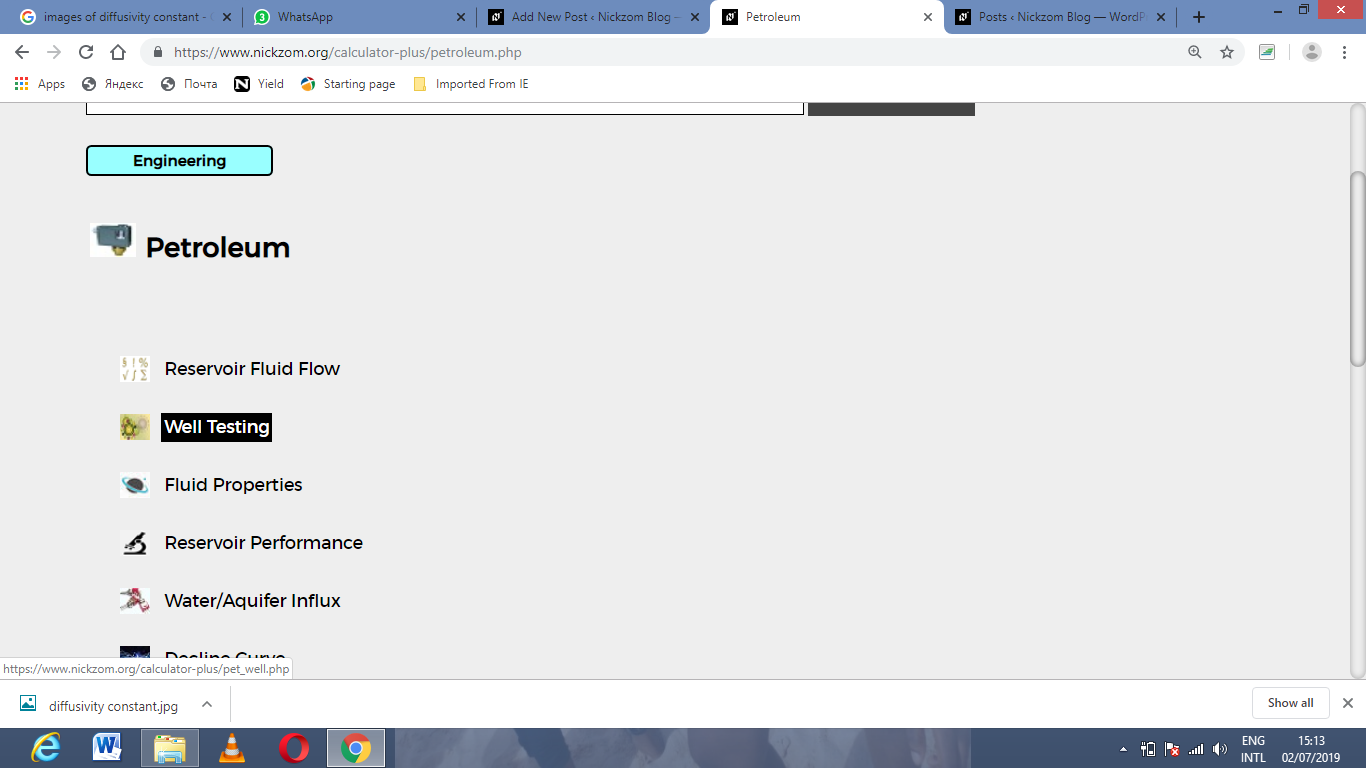Now, Click on Dimensionless Time under Well Testing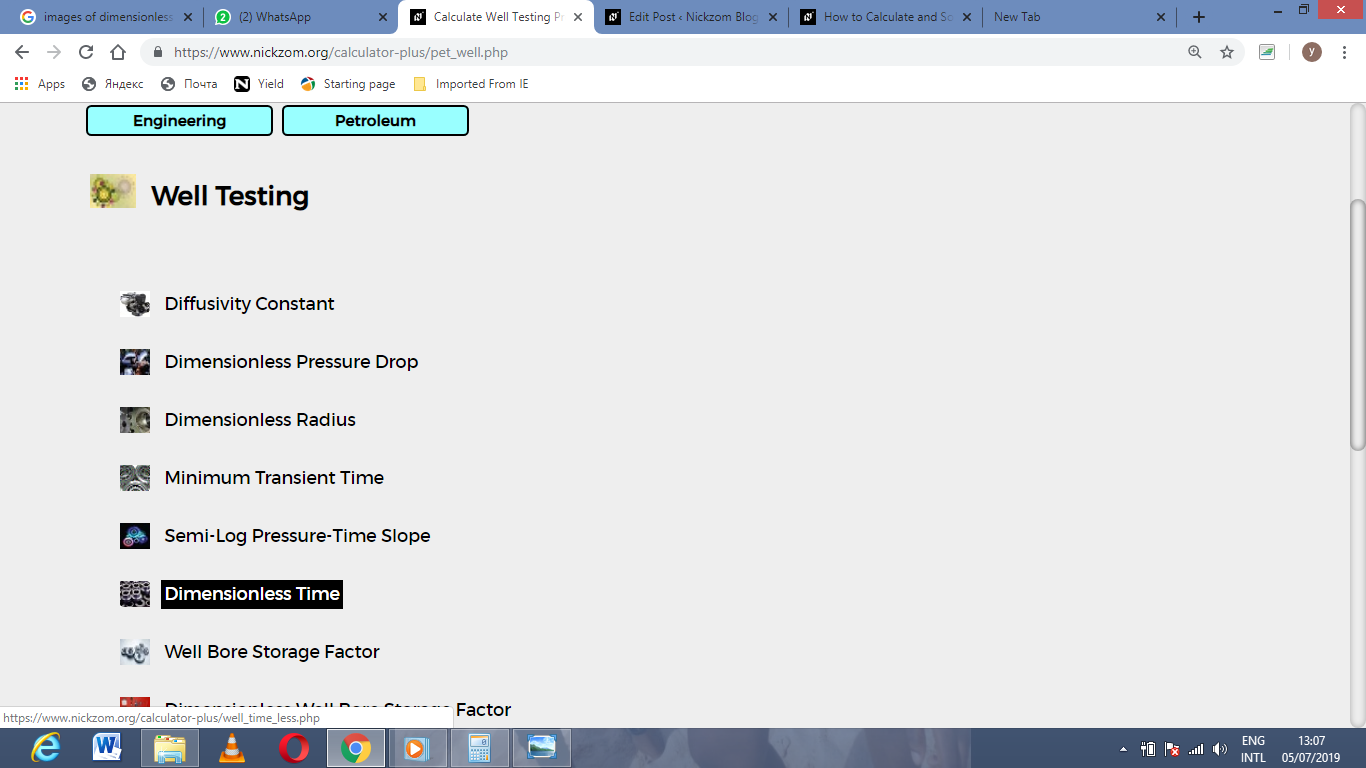The screenshot below displays the page or activity to enter your value, to get the answer for the dimensionless time according to the respective parameter which are the permeability (k), porosity (φ), viscosity (μ), well radius (rw), minimum transient time (t) and total compressibility (CT).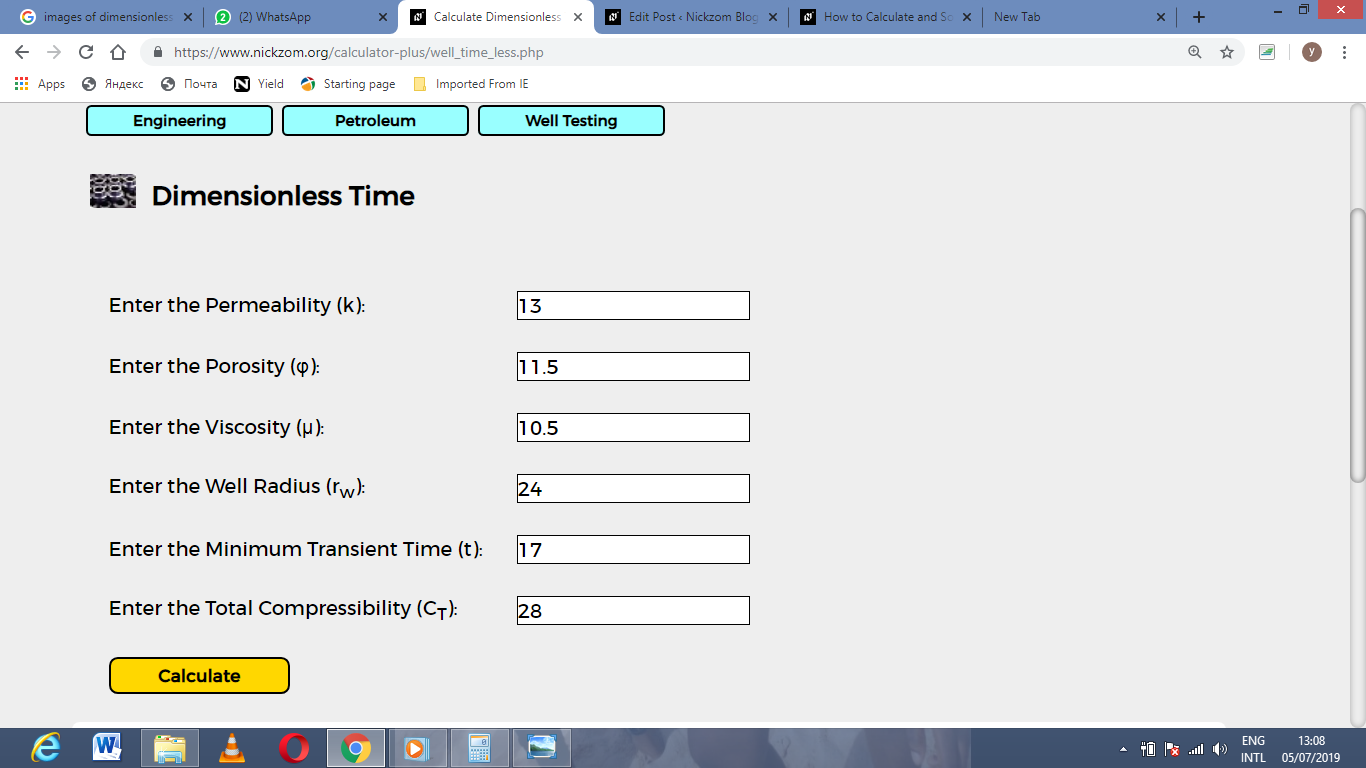Now, enter the value appropriately and accordingly for the parameter as required by the permeability (k) is 13, porosity (φ) is 11.5, viscosity (μ) is 10.5, well radius (rw) is 24, minimum transient time (t) is 17 and total compressibility (CT) is 28.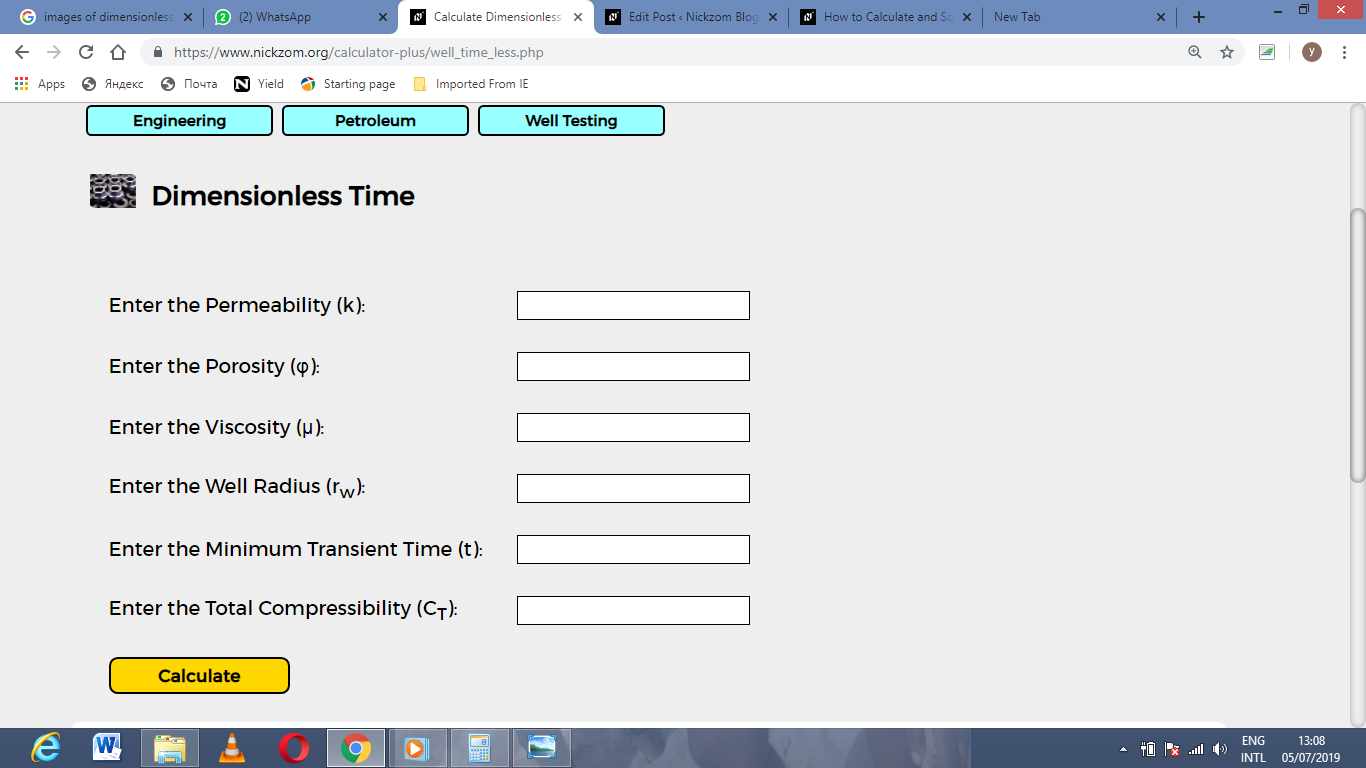Finally, Click on Calculate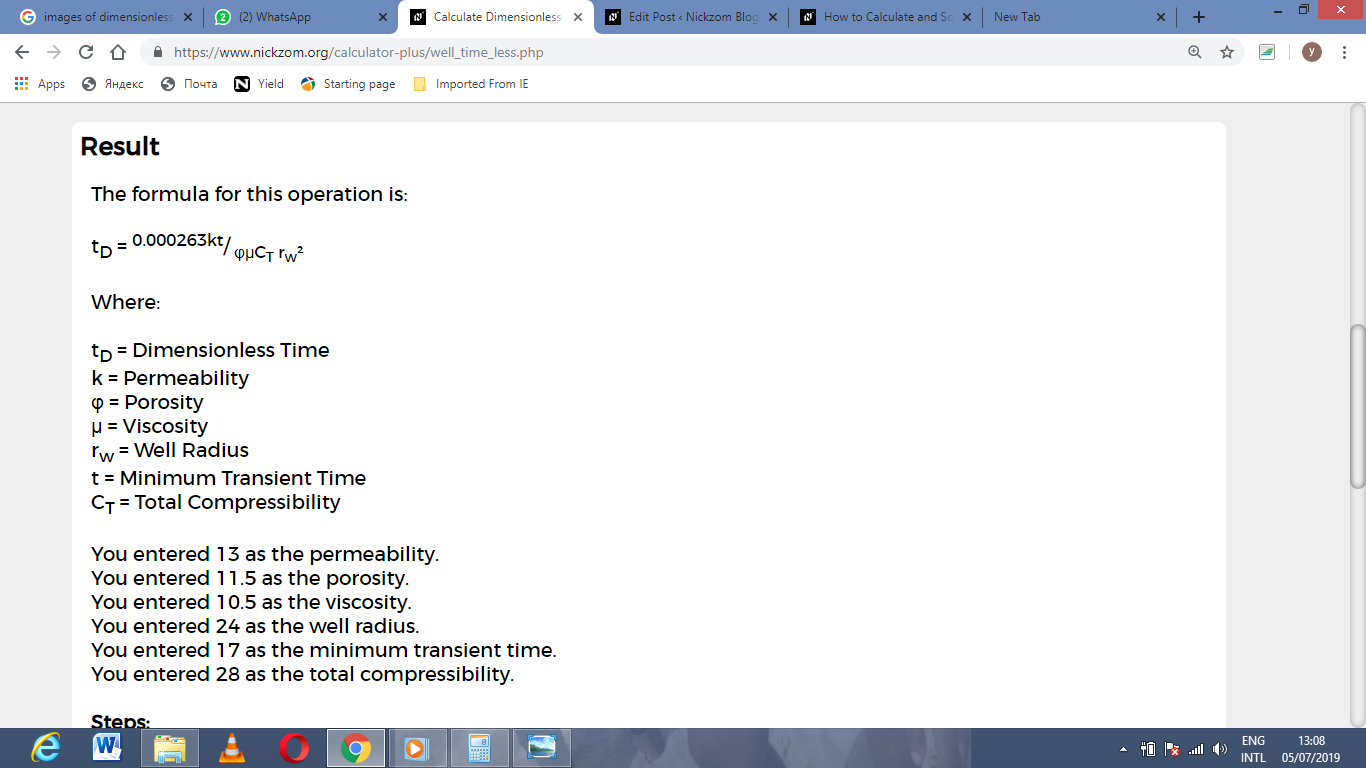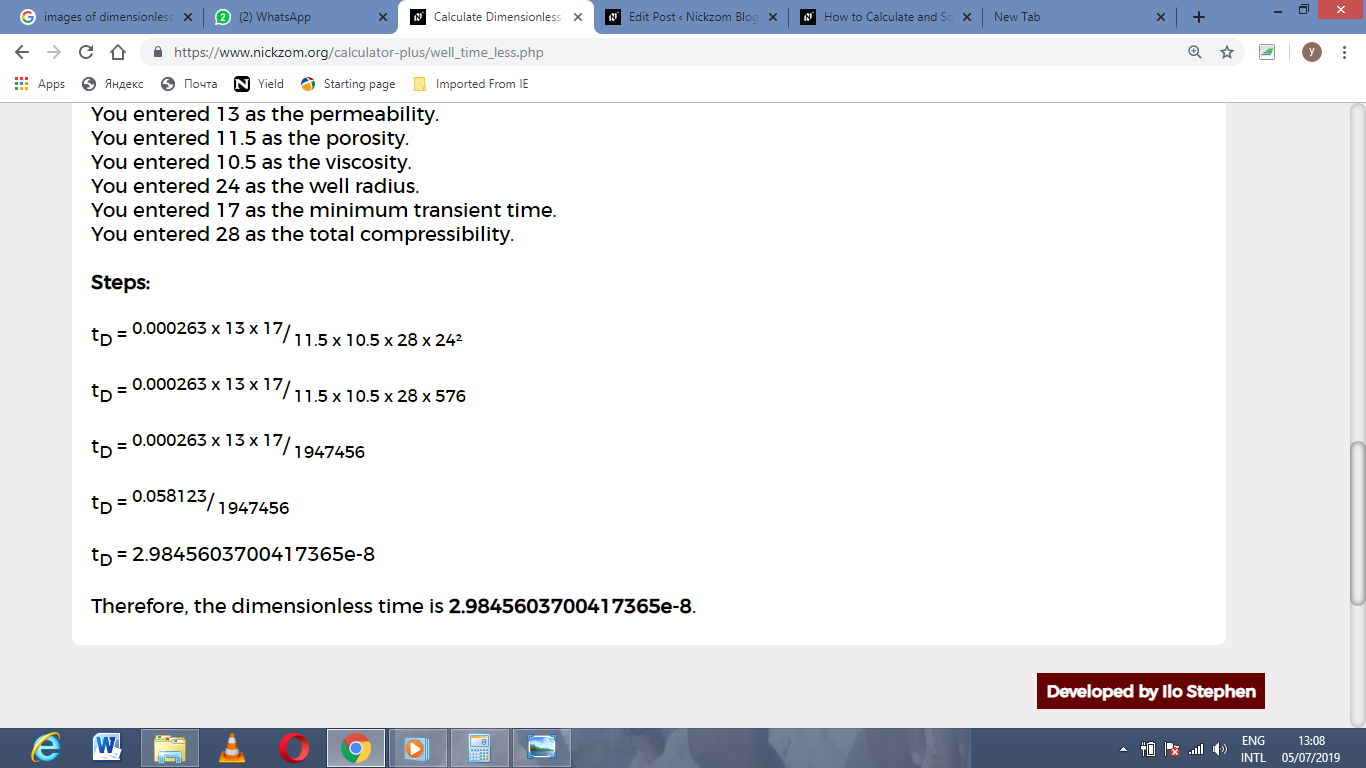As you can see from the screenshot above, Nickzom Calculator– The Calculator Encyclopedia solves for the dimensionless time and presents the formula, workings and steps too.

How to Calculate and Solve for Minimum Transient Time in Well Testing | The Calculator Encyclopedia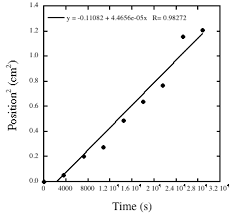The image above represents minimum transient time.

To compute for the minimum transient time, five essential parameters are needed and these parameters are permeability (k), porosity (φ), reservoir radius (r), viscosity (μ) and total compressibility (CT).

The formula for calculating the minimum transient time:

t = 9.48 x 104 φ CT r² / k

Where;

t = Minimum Transient Time
k = Permeability
φ = Porosity
r = Reservoir Radius
μ = Viscosity
CT = Total Compressibility

Let’s solve an example;
Given that the permeability is 11, porosity is 14, reservoir radius is 7, viscosity is 13 and total compressibility is 22.
Find the minimum transient time?

This implies that;

k = Permeability = 11
φ = Porosity = 14
r = Reservoir Radius = 7
μ = Viscosity = 13
CT = Total Compressibility = 22

t = 9.48 x 104 φ CT r² / k
t = 9.48 x 104 x 14 x 22 x 7² / 11
t = 9.48 x 104 x 14 x 22 x 49 / 11
t = 1430721600 / 11
t = 130065600

Therefore, the minimum transient time is 13006500.

How to Calculate and Solve for Diffusivity Constant | Well Testing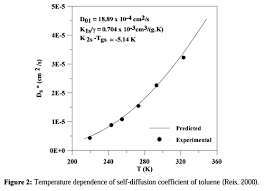The image above represents diffusivity constant.

To compute for the diffusivity constant, four essential parameters are needed and these parameters are pemerability (k), porosity (φ), viscosity (μ) and total compressibility (CT).

The formula for calculating diffusivity constant:

η = 0.000263k / φμCT

Where;

η = Diffusivity Constant
k = Permeability
φ = Porosity
μ = Viscosity
CT = Total Compressibility

Let’s solve an example;
Find the diffusivity constant where the permeability is 12, porosity is 9, viscosity is 7 and total compressibility is 11.

This implies that;

k = Permeability = 12
φ = Porosity = 9
μ = Viscosity = 7
CT = Total Compressibility = 11

η = 0.000263k /  φμCT
η = 0.000263 x 12 / 9 x 7 x 11
η = 0.003156 / 693
η = 0.000004554112554112554

Therefore, the diffusivity constant is 0.000004554112554112554.

Calculating the Permeability when the Diffusivity Constant, Porosity, Viscosity and Total Compressibility is Given.

k = n x φμCT / 0.000263

Where;

k = Permeability
η = Diffusivity Constant
φ = Porosity
μ = Viscosity
CT = Total Compressibility

Let’s solve an example;
Find the permeability where the diffusivity constant is 24, porosity is 10, viscosity is 5 and total compressibility is 13.

This implies that;

η = Diffusivity Constant = 24
φ = Porosity = 10
μ = Viscosity = 5
CT = Total Compressibility = 13

k = n x φμCT / 0.000263
k = 24 x 10 x 5 x 13 / 0.000263
k = 15600 / 0.000263
k = 59315589.3536

Therefore, the permeability is 59315589.3536.

Calculating the Porosity when the Diffusivity Constant, Permeability, Viscosity and Total Compressibility is Given.

φ = 0.000263k / nμCT

Where;

φ = Porosity
k = Permeability
η = Diffusivity Constant
μ = Viscosity
CT = Total Compressibility

Let’s solve an example;
Find the porosity where the diffusivity constant is 28, permeability is 8, viscosity is 12 and total compressibility is 18.

This implies that;

k = Permeability = 8
η = Diffusivity Constant = 28
μ = Viscosity = 12
CT = Total Compressibility = 18

φ = 0.000263k / nμCT
φ = 0.000263 x 8 / 28 x 12 x 18
φ = 0.002104 / 6048
φ = 3.478e-7

Therefore, the porosity is 3.47e-7.

How to Calculate and Solve for Solution Gas – Oil Ratio of a Reservoir Fluid Flow | The Calculator Encyclopedia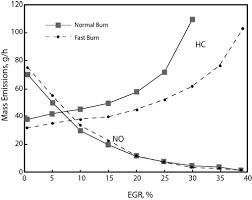The image above represents a Gas – Oil Ratio.

To compute for the gas – oil ratio, seven essential parameters are needed and these parameters are Solution Gas (Rs), Oil Viscosity (μo), Gas Viscosity (μg), Relative Permeability to Gas (krg), Relative Permeability to Oil (kro), Gas Formation Volume Factor (Bg) and Oil Formation Volume Factor (Bo).

The formula for calculating the gas – oil ratio:

GOR = Rs + [krg /  kro] [μoBo / μgBg]

Where;

GOR = Solution Gas-Oil Ratio
Rs = Solution Gas
μo = Oil Viscosity
μg = Gas Viscosity
krg = Relative Permeability to Gas
kro = Relative Permeability to Oil
Bg = Gas Formation Volume Factor
Bo = Oil Formation Volume Factor

Let’s solve an example;
Find the Gas – Oil Ratio with a solution gas of 11, oil viscosity of 7, gas viscosity of 9, relative permeability to gas of 12, relative permeability to oil of 16, gas formation volume factor of 22 and oil formation volume factor of 18.

This implies that;

Rs = Solution Gas = 11
μo = Oil Viscosity = 7
μg = Gas Viscosity = 9
krg = Relative Permeability to Gas = 12
kro = Relative Permeability to Oil = 16
Bg = Gas Formation Volume Factor = 22
Bo = Oil Formation Volume Factor = 18

GOR = Rs + [krg /  kro] [μoBo / μgBg]
GOR = 11 + [12/16] [7 x 18 /9 x 22]
GOR = 11 + [12/16] [126/198]
GOR = 11 + [12/16] [0.63]
GOR = 11 + [0.75] [0.63]
GOR = 11 + 0.477
GOR = 11.477

Therefore, the Gas – Oil Ratio is 11.477.

How to Calculate and Solve for Radial Flow Rate in Reservoir Fluid Flow | The Calculator Encyclopedia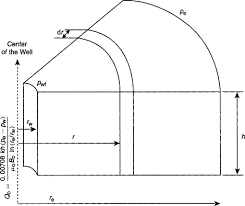The image above represents the radial flow rate.

To compute for the radial flow rate, eight essential parameters are needed and these parameters are External Pressure (Pe), Flowing Bottom-Hole Pressure (Pwf), Formation Thickness (h), Oil Viscosity (μo ), Permeability (k), Oil Formation Volume Factor (Bo), Drainage Radius (reand Well Bore Radius (rw ).

The formula for calculating the radial flow rate:

Qo = 0.00708kh[Pe – Pwf] / μo Bo In[re / rw]

Where;

Qo = Radial Flow Rate
Pe = External Pressure
Pwf = Flowing Bottom-Hole Pressure
h = Formation Thickness
μo = Oil Viscosity
k = Permeability
Bo = Oil Formation Volume Factor
re = Drainage Radius
rw = Well Bore Radius

Let’s solve an example;
Find the radial flow rate when the External Pressure is 14, Flowing Bottom-Hole Pressure is 21, Formation Thickness is 7, Oil Viscosity is 35, Permeability is 50, Oil Formation Volume Factor is 13, Drainage Radius is 26 and Well Bore Radius is 15.

This implies that;

Pe = External Pressure = 14
Pwf = Flowing Bottom-Hole Pressure = 21
h = Formation Thickness = 7
μo = Oil Viscosity = 35
k = Permeability = 50
Bo = Oil Formation Volume Factor = 13
re = Drainage Radius = 26
rw = Well Bore Radius = 15

Qo = 0.00708kh[Pe – Pwf] / μo Bo In[re / rw]
Qo = 0.00708 x 50 x 7 [14 – 21] / 35 x 13 In[26 / 15]
Qo = 0.00708 x 50 x 7 [-7] / 35 x 13 In[26 / 15]
Qo = 0.00708 x 50 x 7 [-7] / 35 x 13 In[1.73]
Qo = 0.00708 x 50 x 7 [-7] /35 x 13 x 0.55
Qo = 2.478 [-7] / 35 x 13 x 0.55
Qo = -17.346 / 250.27
Qo = -0.069

Therefore, the radial flow rate is -0.069 STB/day.

How to Calculate and Solve for Linear Flow Rate in Reservoir Fluid Flow | The Calculator Encyclopedia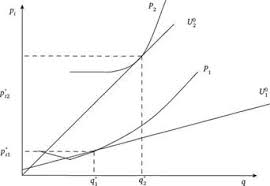The image above represents the linear flow rate.

To compute for the linear flow rate, four parameters are needed and these parameters are Initial pressure (P1), Final Pressure (P2), Thickness (h) and Permeability (k).

The formula for calculating linear flow rate:

q = 0.001127kh[P1 – P2]

Where:
q = Linear Flow Rate
P1 = Initial Pressure
P2 = Final Pressure
h = Thickness
k = Permeability

Let’s solve an example;
Find the linear flow rate when the initial pressure is 12, final pressure is 22, thickness is 18 and permeability is 44.

This implies that;
P1 = Initial Pressure = 12
P2 = Final Pressure = 22
h = Thickness = 18
k = Permeability = 44

q = 0.001127kh [P1 – P2]
q = 0.001127 x 44 x 18 [12 – 22]
q = 0.001127 x 44 x 18 [-10]
q = 0.892584 [-10]
q = -8.92584

Therefore, the linear flow rate is -8.92584 bbl/day.

Calculating Permeability when the linear flow rate, Initial Pressure, Final Pressure and Thickness is Given.

k = q / 0.001127h (p1 – p2)

Where;
k = Permeability
q = Linear Flow Rate
P1 = Initial Pressure
P2 = Final Pressure
h = Thickness

Let’s solve an example;
Find the permeability when the initial pressure is 34, final pressure is 24, thickness is 12 and linear flow rate is 50.

This implies that;
q = Linear Flow Rate = 50
P1 = Initial Pressure = 34
P2 = Final Pressure = 24
h = Thickness = 12

k = q / 0.001127h (p1 – p2)
k = 50 / 0.001127h (34 – 24)
k = 50 / 0.001127h (10)
k = 50 / 0.01127
k = 4436.5

Therefore, the permeability is 4436.5.